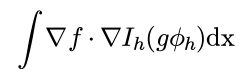# How to set up a linear form with an interpolation operator

Dear all,
I’m trying to set up the following linear form in NGSolve (see the image in the attachment) where f and g are two known functions and \phi_h is my test. But the problem is that I have no idea how to implement the Lagrange interpolation I_h in the code. Does anybody have some ideas? Thank you very much!Hi,

the Interpolate command interpolates a given function into a given finite element space

Grad(f)*Grad(Interpolate(g*phi_h, H1(mesh,order=1)))*dx


Best,
Michael